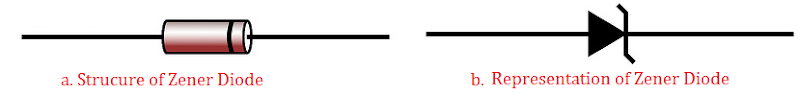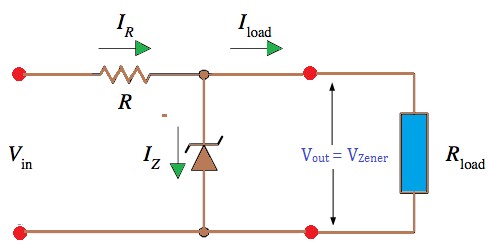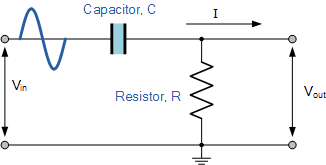# Zener Diode and Filter Circuit

Zener diode

Zener diode is an electronic component that allows the flow of current in only one direction. It is the properly crystal diode which works in reverse breakdown region. The representation of zener diode is given below.Zener diode and its representation

Zener Diode as a voltage regulator:Zener Diode as a voltage regulator

Zener diode works in the breakdown region. So, voltage across it is nearly constant even though current through it changes when it works in the breakdown region. In the given figure, a zener diode D is connected in series with the resistance R and variable input Vin which is generally the output voltage from a rectifier. D is reversed biased so that the it works in the breakdown region. The sharp breakdown voltage is called zener voltage (Vz). When the input voltage Vin increases and exceeds the zener voltage, the diode gives constant output voltage and Vz and rest of the voltage is dropped across R. When the input voltage decreases (or equals to that of Vz), a small voltage drop takes place across R and the output voltage is still Vz. Then we get a constant power voltage in spite of fluctuating voltage. Hence zener diode acts as a voltage regulator.

Filter Circuit: The device which allows only DC components to reach the load by removing any AC component is known as filter circuit. A filter circuit is needed to get steady voltage with minimum ripple.Circuit diagram for filter circuit

The filter circuit consists of passive elements like inductor, capacitor and a resistor on their combination. There are four major types of filter  circuits. Types of filter  circuit are  described and studied briefly in higher level. Here we mention only the types of filter circuit.

1. Capacitor filter circuit
2. Inductor filter circuit
3. LC filter circuit
4. Pi (π) filter circuit

Do you like this article ? If yes then like otherwise dislike : 1